# Pseudo-Euclidean space

A real affine space in which to any vectors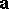and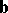there corresponds a definite number, called the scalar product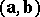(cf. also Inner product), satisfying

1) the scalar product is commutative: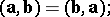2) the scalar product is distributive with respect to vector addition: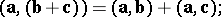3) a scalar factor can be taken out of the scalar product:4) there existvectors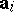such thatThe numberis called the dimension of the pseudo-Euclidean space,is called the index, the pair of numbers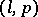,, is called the signature. A pseudo-Euclidean space is denoted by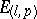(or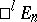). The space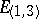is called the Minkowski space. In any system ofvectors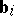infor which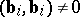and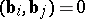when, the number of vectorsfor which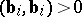is equal toand the number of vectorsfor which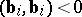is equal to(the law of inertia for a quadratic form).

The modulusof a vectorin a pseudo-Euclidean space can be defined as the non-negative root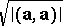. The vectors that have scalar squares equal to 1 or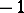are called unit and pseudo-unit vectors, respectively. The vectors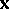for which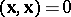have zero modulus and are called isotropic vectors. The directions of the isotropic vectors are the isotropic directions.

In a pseudo-Euclidean space there are three types of straight lines: Euclidean, having direction vector with positive scalar square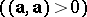, pseudo-Euclidean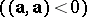and isotropic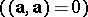. The union of all the isotropic straight lines passing through a certain point is called the isotropic cone.

In a pseudo-Euclidean space there are several types of planes: Euclidean planes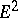, pseudo-Euclidean planes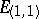and planes containing isotropic vectors, the so-called semi-Euclidean planes with signaturesand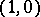and deficiency 1 (see Semi-Euclidean space) and isotropic planes, all vectors of which are isotropic.

The distance between two points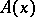and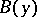is taken to be the modulus of the vectorand is computed from: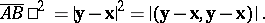A pseudo-Euclidean space is not a metric space, since the triangle inequality is not satisfied. If the vectorsandbelong to a Euclidean plane (or to a pseudo-Euclidean plane of index 0), then they satisfy the triangle inequality, but if they belong to a pseudo-Euclidean plane of index 1, then they satisfy the so-called inverse triangle inequality:In a pseudo-Euclidean space there are three types of spheres: spheres with positive radius squared,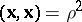, spheres with negative radius squared,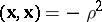, and spheres of zero radius,, which are just the isotropic cones.

The motions of a pseudo-Euclidean space are affine transformations (cf. Affine transformation) and can be written in the form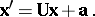The operator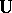satisfies the condition, that is, it preserves distances between points. The motions of a pseudo-Euclidean space form a multiplicative group; it depends on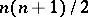independent parameters. The motions of a pseudo-Euclidean space are called motions of the first or second kind if they are affine transformations of the corresponding kind.

Geometric transformations are called anti-motions when each vectorgoes to a vector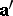for which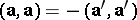.

The basic operations of vector and tensor algebra can be introduced into a pseudo-Euclidean space. The basic differential-geometric concepts are constructed in accordance with the rules of the geometry of pseudo-Riemannian space. The metric tensor of a pseudo-Euclidean space has the form (in a Galilean coordinate system)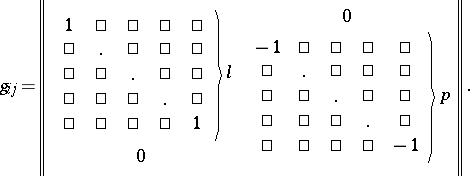A pseudo-Euclidean space is flat, that is, its Riemann tensor is zero. If the Riemann tensor of a pseudo-Riemannian space is identically zero, then it is a locally pseudo-Euclidean space.

Subsets of a pseudo-Euclidean space can carry various metrics: A positive- or negative-definite Riemannian metric, a pseudo-Riemannian metric or a degenerate metric (see Indefinite metric). For example, the spheres of a pseudo-Euclidean space carry a (generally speaking, indefinite) metric of constant curvature. In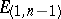a sphere with positive radius squared is an-dimensional space isometric to the hyperbolic space.

The pseudo-Euclidean space(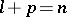) and the Euclidean space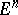can be considered as subspaces of a complex space with form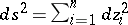. If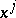are coordinates in the pseudo-Euclidean space,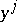are those of the real Euclidean space and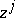those of the complex Euclidean space, then the equations of the subspaces have the form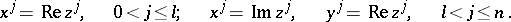The metric of the pseudo-Euclidean space can be formally obtained from the metric of the Euclidean space by the substitution,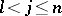.